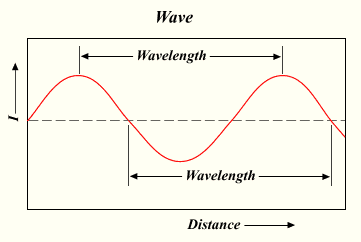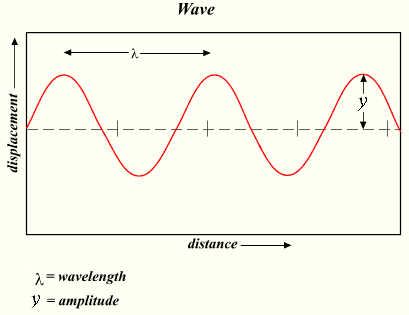# Sine wave facts for kids

Kids Encyclopedia Facts

A sine wave is a curve with this shape:This is a picture of a sine wave.

All waves can be made by adding up sine waves. The sine wave has a pattern that repeats. The length of this repeating piece of the sine wave is called the wavelength. The wavelength can be found by measuring the length or distance between one peak of a sine wave and the next peak. The wavelength can be found in many other ways too.

## Waves made of Sine Waves

Waves are found everywhere in the natural world. Examples of waves:

All of these waves are sums of signals.

## Properties of Sine Waves

Sine waves have a "length" called a wavelength. There are other properties of waves and sine waves, such as their frequency, amplitude, phase, and speed.

The frequency of a sine wave is the number of oscillations a wave has in a certain period of time, for example one second.## Images for kidsSine wave Facts for Kids. Kiddle Encyclopedia.